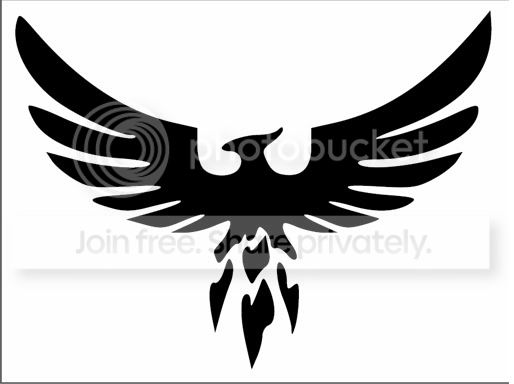MAIN: Orange; - default.html - x1.css -This page = Hello World - (something to upload, .. and test functionality - while other NEW pages -/- content is assembled)

k

k

k

k

k

k

k

k

k

k

k

k

k

k

k

k

k

k

k

k

k

k

k

k

k

k

k

k

k

k

k

k

k